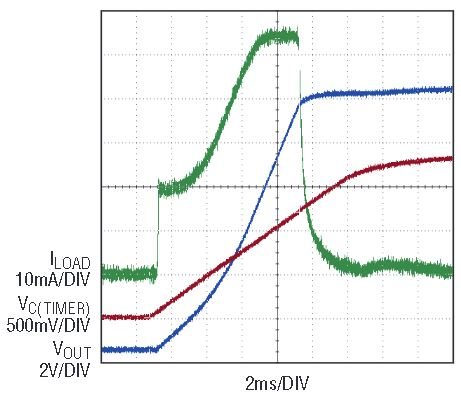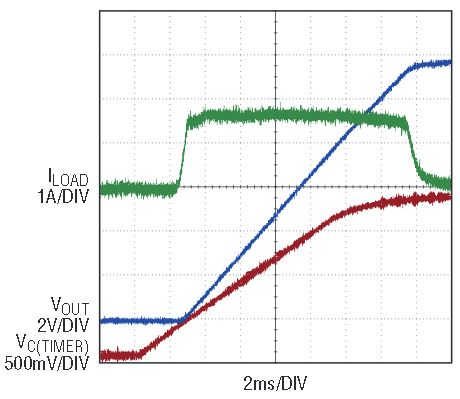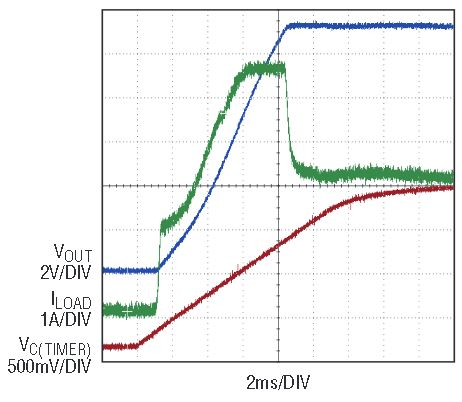# Analysis of Hot Swap Circuits with Foldback Current Limit

A reliable analog circuit guarantees proper operation within the parametric tolerances of the active controlling IC and passive components. For a Hot Swap circuit to perform properly, the minimum and maximum values of a number of parameters must be gathered from the data sheets of all components. From these, the Hot Swap circuit’s behavior in the face of various capacitive loads should be known. This article shows how critical capacitive loads are calculated for Hot Swap circuits with foldback current limit characteristics.

Overview

For a Hot Swap circuit, as shown in Figure 1, the critical parameters are operating voltage (VOPER) maximum current limit (ILIMIT) timer period (T) and the maximum output voltage slew rate (SO), which happens when the Hot Swap circuit starts to operate with no-load. These parameters are selected initially based on the load requirements, supply limitations and MOSFET’s drain-source on-resistance (RDS(ON)) and its safe operating area (SOA).Figure 1. (a) Major functional components of a Hot Swap circuit and (b) linear approximation of the foldback characteristic.

While slewing, the MOSFET acts as a source follower so the maximum output voltage slew rate, SO, is the same as the GATE pin slew rate SG, and it is defined by the circuit components (gate current divided by gate to ground capacitance), and has a strong influence on the power up transient. The timer period, T, is the time allowed for the Hot Swap circuit to operate in current limit mode before a fault is generated. A successful power-up transient is one that does not generate a fault.

The problem of proper operation over the full variation of the circuit parameters is relatively simple for a circuit with constant current limit, ILIMIT. The relationship of parameters of a purely capacitive load at the constant current limit for time T is:

For an R-C load it is easy to define a surplus current, which is allowed for the capacitive component of the load, and select proper load capacitance.

There are two common problems that require solutions when charging a load with a Hot Swap circuit:

• The maximum pure capacitive load for a successful power-up transient.
• The maximum capacitive load, which can be added in parallel to a resistive load, RL, for a successful power-up transient.

A linear approximation of the foldback characteristic is shown in Figure 1b. It is used in all the following considerations. The main points of this characteristic are:

• The operating voltage is VOPER.
• The initial current limit value, when the output voltage 0 ≤ VOUT ≤ VINIT, is IINIT.

This current limit value persists until the output voltage reaches VLIMIT(point A), after which the current limit increases linearly, which occurs after the output voltage reaches VFIX(point B). After point B the current limit is constant (ILIMIT = constant). The voltage VFIX is lower than VOPER.

The current limit value as a function of the output voltage, shown in Figure 1b, is expressed by three separate equations for different output voltage levels:

The value of the parameters marked on Figure 1b, the timer period T value and the slew rate SO are all known, with tolerance, and used in the following solutions. For some circuits, the slew rate SO is fast enough that it has a negligible effect on the inrush transient, and for others, it is significant. The two loads above are solved for these two cases of slew rate.

Calculating the Maximum Pure Capacitive Load

One important parameter to know for a Hot Swap circuit is the maximum pure capacitive load that a circuit can successfully power up into without a fault.

Consider two critical capacitive loads: CNO_ FLT and CFLT. CNO_ FLT is the maximum capacitive load with which the circuit passes the power-up transient without a fault for any possible combination of circuit parameters. CFLT is the minimum capacitive load with which the power-up transient is always unsuccessful, and a fault is generated. From these, the capacitive load range can be divided into three groups. The power-up transient is successful for capacitive loads from zero to CNO_ FLT. Power-up is unsuccessful for loads larger than CFLT. The power-up transient is unpredictable for loads from the CNO_ FLT to CFLT.

The following Hot Swap circuit parameters can be initially defined with tolerance: VINIT, VFIX, IINIT, ILIMIT, T.

The function ILOAD(VOUT) shown in Figure 1b and equations (1–3) has three distinct operating regions for current limit. The slew rate, SO, can either cause the circuit to leave a current limit mode in any of these operating regions (before the timer period T expires), or it can have no effect (i.e., SO is very fast). Each of these scenarios, transients, should by analyzed for any Hot Swap circuit. Each is described below. Some transients allow finding an analytical expression for the worst-case parameters.

However, the general or universal solution can be obtained in a numerical form.

Case 1: SO Never Limits Current

Suppose that natural slew rate SO is fast enough to keep the operating point in current limit mode in all three portions of the function ILOAD(VOUT).

In the first stage of the transient, the current is IINIT and the output voltage rises linearly from zero to VINIT during the time t1. The capacitive load CLOAD1 can be expressed as:

In the second stage of the transient, the current increases linearly from IINIT to ILIMIT according to (2) as the output voltage increases from VINIT to VFIX. Time t2 represents the duration of this stage, completed when the output voltage reaches VFIX. VFIX is usually set to (0.5 to 0.9) VOPER (it must be lower than VOPER) by proper selection of the resistive divider.

The output voltage as a function of time is:

Substituting the expression (2) in equation (5) produces:

or

which leads to the first order differential equation

with initial condition

Equation (8) describes VOUT(t) from the start of stage 2, t = 0, to time t2, when the current limit reaches its maximum value, ILIMIT.

The solution for Equation (8) is:

The output voltage at time t2 is VFIX

The duration of interval t2 is

The third equation should describe how CLOAD1 is charged with current ILIMIT during the interval t3 from VFIX to some intermediate-level VINTERIM where the operation point leaves a current limit mode because the MOSFET transconductance drops off in the triode region. When this region is entered, successful start-up is assured, but the point at which this region begins is difficult to solve for because it involves MOSFET parameters that may not be available. This region is usually small, so it makes sense to simplify the description of this region with the assumption that CLOAD1 is charged from VFIX to VOPER with ILIMIT. In this case the time:

according to (4)

Taking into account T = t1 + t2 + t3, the capacitive load could be expressed

The minimum value of CLOAD1 from equation (15) is obtained with TMIN, VINIT_MAX, IINIT_MIN, and VFIX_MAX. The same parametric limits must be used for expression of CNO_FLT that follows.

Figure 2 shows this type of start-up transient.Figure 2. (Case 1) Pure capacitive load. Operating point leaves current limit mode in the third area, where current limit is ILIMIT.

The output voltage slew rate range for this case falls in the range:

Case 2: SO Limits Current at Point A

For the case where the slew rate limit, SO, causes the operating point to leave a current limit mode exactly at point A in Figure 1, the capacitive load CLOAD2 can be expressed as:

CLOAD2 has a minimum with IINIT_MIN, TMIN and VINIT_MAX.

It should be noted that the maximum output voltage slew rate in this case is

It is constant while the operating point resides in the current limit mode.

Figure 3 shows this transient.Figure 3. (Case 2) Pure capacitive load with a limited SO (natural output voltage slew rate). Operating point leaves current limit mode in the first area, where current limit is IINIT.

Case 3: SO Limits Current at Point B

To produce an analytical expression of CLOAD3 for the case when the operating point leaves a current limit mode at point B, assume that it happens exactly as the output voltage reaches VFIX. The full duration of operation in current limit mode, T, includes two intervals t1 and t2. According to (14) t1 is:

In a second part of this transient, the output voltage changes according to expression (10) and the duration of interval t2 is:

and from (17) and (18)

From equation (19), the minimum value of CLOAD3 is produced using TMIN, VINIT_MAX, IINIT_MIN, and VFIX_MAX.

The output voltage slew rate range for this case can be defined as:

Case 4: SO Limits Current Between Points A and B

If the output voltage slew rate SO is in the range SLOAD1_MIN ≤ SO ≤ SLOAD1_MAX, current limiting stops before the output voltage reaches VFIX (before point B), meaning that during the time t1_4 the output voltage slew rate is a constant (due to IINIT) and during the time t2_4 it becomes equal to SO. The following method is recommended.

The output voltage rises from zero to the VFIX during the time t1_4, which can be expressed from (16) as

The output voltage VOUT(t2_4) should be equal to the voltage on the second stage of the characteristic Figure 1 (equation 2):

Substituting ILOAD(t2_4) in (14) with SO CLOAD4, taking into an account that t2_4 = T − t1_4, and placing the equal sign between (14) and (11) forms the following equation:

This transcendental equation (21) with unknown CLOAD4 can be solved with the proper calculation software (Mathcad, MATLAB, Mathematica) or LTspice.

Figure 4 demonstrates a successful power-up transient, where the current is limited for a time less than the timer period at maximum slew rate. As a result, the transient begins in current limit mode and finishes the inrush in slew rate limited operation.Figure 4. (Case 4) Pure capacitive load with a limited SO (natural output voltage slew rate). Operating point leaves current limit mode in the second area, where current limit rises linearly.

Maximum Capacitive Load for Successful Power-Up Transient without the Limitation of the Output Voltage Slew Rate and a defined Resistive Load

If the passive load can be defined as a resistive load RL and all Hot Swap circuit parameters (VOPER, VFIX, IINIT, ILIMIT, T) are known, then the maximum capacitive load should be found to ensure a successful power-up transient. A successful power-up transient is completed only after the current reaches ILIMIT, because the slew rate is fast enough to stay in a current limit for the entire transient.

The differential equation for the first stage is:

The equation (22) solution is:

At the end of the first stage (t1) the output voltage is equal to VINIT and

The differential equation for the second stage is:

The first component on the left side of equation (25) is a current charging the capacitor; the second one is a resistive current.

The solution to equation (23) describes the output voltage from the beginning of this stage to the time t2, when the output voltage reaches VFIX.

The time interval t2 could be expressed as a function of CLOAD from (26) as:

The differential equation for the third stage is:

With the assumption that the output voltage slew rate, SO, does not affect the transient, it is possible to say that the output voltage is changing according to (28) up to VOPER. The solution for equation (28) is:

At the end of this stage:

And time t3 equals:

Since T = t1 + t2 + t3, CLOAD for this case is:

Figure 5 shows measured results for this case.Figure 5. R-C load without limitation for SO. Operating point leaves current limit mode in the third area, where current limit is ILIMIT.

Maximum Capacitive Load for Successful Power-Up Transient when Current is Limited by the Output Voltage Slew Rate and a Defined Resistive Load is Present

The value of the output voltage slew rate, SO, defines how long a Hot Swap circuit should operate in the current limit mode. This event (leaving the current limit mode) can happen at any moment of the three stages of the transient. For defined points A and B, during the third stage it is possible to use equations from the previous section. For any intermediate points, it is possible to use the approach demonstrated in “Case 4” with a transcendental equation.

The transient in Figure 6 illustrates this case, when the operating point leaves the current limit mode in the first stage of current limit area.Figure 6. R-C load with limited SO. Operating point leaves current limit mode in the first area, where current limit is IINIT.

Conclusion

The derived expressions in this article and the approach to the numerical solutions can serve as a basis for a detailed optimization of Hot Swap solutions.